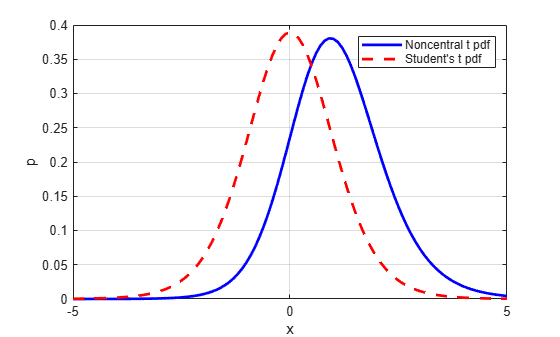# nctpdf

Noncentral t probability density function

## Syntax

```Y = nctpdf(X,V,DELTA) ```

## Description

`Y = nctpdf(X,V,DELTA)` computes the noncentral t pdf at each of the values in `X` using the corresponding degrees of freedom in `V` and noncentrality parameters in `DELTA`. Vector or matrix inputs for `X`, `V`, and `DELTA` must have the same size, which is also the size of `Y`. A scalar input for `X`, `V`, or `DELTA` is expanded to a constant matrix with the same dimensions as the other inputs.

## Examples

collapse all

Compute the pdf of a noncentral t distribution with degrees of freedom `V = 10` and noncentrality parameter `DELTA = 1`. For comparison, also compute the pdf of a t distribution with the same degrees of freedom.

```x = (-5:0.1:5)'; nct = nctpdf(x,10,1); t = tpdf(x,10);```

Plot the pdf of the noncentral t distribution and the pdf of the t distribution on the same figure.

```plot(x,nct,'b-','LineWidth',2) hold on plot(x,t,'g--','LineWidth',2) legend('nct','t')```## References

 Evans, M., N. Hastings, and B. Peacock. Statistical Distributions. 2nd ed., Hoboken, NJ: John Wiley & Sons, Inc., 1993, pp. 147–148.

 Johnson, N., and S. Kotz. Distributions in Statistics: Continuous Univariate Distributions-2. Hoboken, NJ: John Wiley & Sons, Inc., 1970, pp. 201–219.